M340L Practice 3rd Midterm (From November, 1993)

Problem 1: Consider the linear operator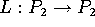,
L(p(t)) = (t+1)p'(t) - 2p(t). As usual,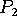is the space of 2nd order polynomials in the variable t.

a) What is the matrix of L relative to the basis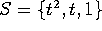?

b) Find the dimension of the kernel of L and the dimension of the range of L.

c) Find a basis for the kernel of L. Also, find a basis for the range of L.

Problem 2 This problem concerns changing bases in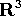. Let S be the standard basis. Let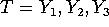be another basis, where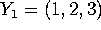,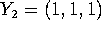,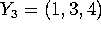.

a) Find a matrix P that converts from the T basis to the S basis. That is, so that for any vector X,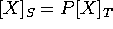.

b) Find a matrix Q that converts from the S basis to the T basis. That is, so that for any vector X,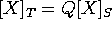.

c) There is a linear operator L whose matrix, relative to the S basis, is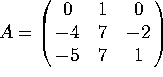. Find the matrix of L relative to the T basis.

Problem 3. Let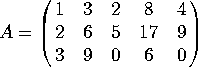a) What is the rank of A?

b) Find a basis for the null space of A.

c) Find a basis for the row space of A.

Problem 4. Let V be the span of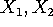and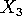, where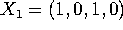,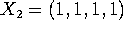and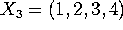.

a) Find an orthogonal basis for V.

b) Find an orthonormal basis for V.

Problem 5. True of False

a) The map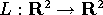, L(x,y)=(x+y,y-1), is a linear transformation.

b) The map,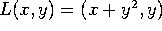, is a linear transformation.

c) The map, L(x,y)=(3 x+ y,y-5x), is a linear transformation.

d) Let L be a linear transformation from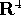to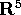. If the rank of L is 3, then the kernel of L is 1-dimensional.

e) Let L be a linear transformation fromto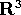. If the kernel of L is 2 dimensional, then L is onto.

f) Let L be a linear transformation fromto. If L is 1-1, then L is onto.

g) If a set of vectors is orthonormal, then it is a basis.

h) If a set of vectors is orthonormal, then it is linearly independent.

i) If a matrix is wider than it is tall (e.g. a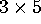matrix), then the row rank is greater than the column rank.

j) Let L be a linear transformation from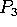to itself. The solutions (in) to the equation L(p)=0 form a vector space.

Fri Apr 4 16:25:02 CST 2003